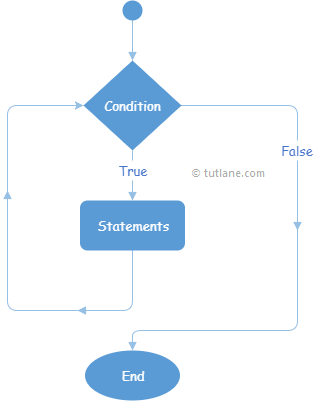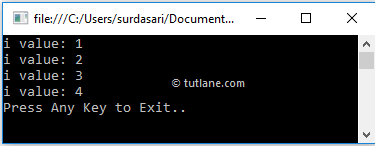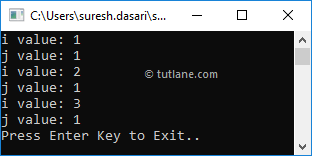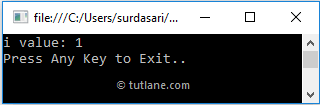# C# While Loop with Examples

In c#, While loop is used to execute a block of statements until the specified expression return as true.

In the previous chapter, we learned about for loop in c# with examples. Generally, the for loop is useful when we are sure about how many times we need to execute the block of statements. If we are unknown about the number of times to execute the block of statements, then while loop is the best solution.

## Syntax of C# While Loop

Generally, while keyword is used to create a while loop in c# applications. Following is the syntax of defining a while loop in c# programming language to execute the block of statements until the defined condition evaluates as false.

while (boolean_expression) {
// Statements to Execute
}

If you observe the above syntax, we used a while keyword to define a while loop, and it contains a parameter called boolean_expression.

Here if boolean_expression returns true, then the statements inside of while loop will be executed. After executing the statements, the boolean_expression will be evaluated to execute the statements within the while loop.

In case the boolean_expression is evaluated to false, then the while loop stops execution of statements, and the program comes out of the loop.

## C# While Loop Flow Chart Diagram

Following is the pictorial representation of while loop process flow in c# programming language.Now we will see how to use while loop in c# programming language with examples.

## C# While Loop Example

Following is the example of using a while loop in c# programming language to execute the block of statements based on our requirements.

using System;

namespace Tutlane
{
class Program
{
static void Main(string[] args)
{
int i = 1;
while (i <= 4)
{
Console.WriteLine("i value: {0}", i);
i++;
}
Console.WriteLine("Press Enter Key to Exit..");
}
}
}

If you observe the above example, we are executing the statements within the while loop by checking the condition (i <= 4) and increasing the variable i (i++) value to 1 by using the increment operator.

When we execute the above c# program, we will get the result as shown below.If you observe the above result, while loop has been executed until it matches the defined condition (i <= 4) and the program came out of the loop whenever the defined condition returns false.

## C# Nested While Loop

In c#, we can use one while loop within another while loop to implement applications based on our requirements.

Following is the example of implementing nested while loop in c# programming language.

using System;

namespace Tutlane
{
class Program
{
static void Main(string[] args)
{
int i = 1;
while (i < 4)
{
Console.WriteLine("i value: {0}", i);
i++;
int j = 1;
while (j < 2) {
Console.WriteLine("j value: {0}", j);
j++;
}
}
Console.WriteLine("Press Enter Key to Exit..");
}
}
}

If you observe the above example, we used one while loop within another while loop to achieve nested while loop functionality in our application based on our requirements.

When we execute the above c# program, we will get the result as shown below.If you observe the above example, both while loops got executed and returned the result based on our requirements.

## C# While Loop with Break Statement

In c#, we can exit or terminate the execution of a while loop immediately by using a break keyword.

Following is the example of using the break keyword in a while loop to terminate the loop's execution in the c# programming language.

using System;

namespace Tutlane
{
class Program
{
static void Main(string[] args)
{
int i = 1;
while (i < 4)
{
Console.WriteLine("i value: {0}", i);
i++;
if (i == 2)
break;
}
Console.WriteLine("Press Enter Key to Exit..");
}
}
}

If you observe the above example, whenever the variable (i) value becomes 2, we terminate the loop using the break statement.

When we execute the above c# program, we will get the result as shown below.This is how we can use break statements with a while loop to terminate loops' execution based on our requirements.